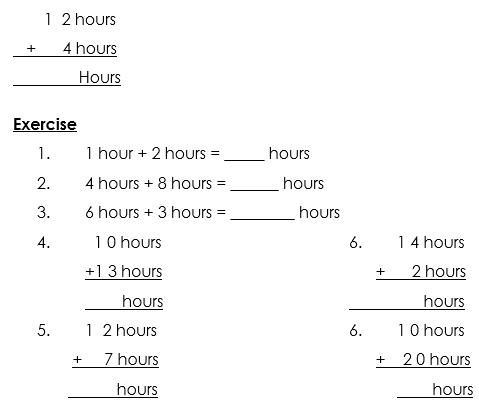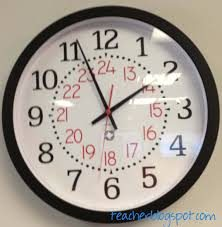SUBTRACTION AND ADDITION OF HOURS-P.1

This unit is about addition and subtraction of hours.it also involved filling in the missing numbers

Addition of time in full hours

Example

3 hours + 8 hours = ____________ hours

3 hours + 4 hours = ___________ hours.Lesson 1

subtraction in full hours

1. 13 hours – 4 hours = __________ hours
2. 10 hours – 3 hours = __________ hours
3. 7 hours – 1 hour = _________ hours
4. 8 hours – 6 hours = ________ hours
5. 4 hours – 4 hours = _________ hours
6. 1 3 hours-5hours  = _________hours

Lesson 3

Finding missing numbers

SEE ALL
•YOU